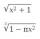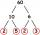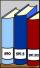# Expression plus minus

Evaluate expression: (-1)2 . 12 – 6 : 3 + (-3) . (-2) + 22 – (-3) . 2

Result

x =  8

#### Solution:Leave us a comment of example and its solution (i.e. if it is still somewhat unclear...):

Showing 0 comments:Be the first to comment!## Next similar examples:

1. With bracketCalculate (evaluate) simple mathematical expression with a negative numbers and a bracket: 13+15*5-2*(-6)
2. ValueDetermine the value of the following exspressions: a) (23-25)·(4-5) b) (97-123):(18+8)
3. Expression 1What is 7+8-(5×2)+5-4+(6×(5-3)+6)-(8+10)-7+6?
4. EvaluateThe division of numbers 18 and 6 increase by product of the numbers 156 and 0.001
5. NumberCalculate the integer number which, divided by 34 gives 10 and the rest 25.
6. Decimal expansionCalculate: 2 . 1 + 0 . 10 + 7 . 10000 + 4 . 1000 + 6 . 100 + 0 . 100000 =
7. ProductResult of the product of the numbers 1, 2, 3, 1, 2, 0 is:
8. If youIf you travel to work 22 days and it takes 29.2 minutes, how many minutes will it take to travel to work and back?
9. Conference 29 people attended a conference on behalf of their company. The conference fee was £520 per person and the company paid a total of £856 in travel costs for the 9 people. How much did the conference cost the company altogether?
10. ConcertOn a Concert were sold 150 tickets for CZK 360, 235 tickets for 240 CZK and 412 for 180 CZK. How much was the total revenues for tickets?
11. The resultHow many times I decrease the number 1632 to get the result 24?
12. DoctorsIn the city operates 171 doctors. The city has 128934 citizens. How many citizens are per one doctor?
13. Math classificationIn 3A class are 27 students. One-third got a B in math and the rest got A. How many students received a B in math?
14. Thermometerthermometer showed -12 degrees Celsius at the morning then the temperature rises by 4 degrees and later again increased by 2 degrees at the evening has fallen by 5 degrees and then fall 3 degrees. What end temperature does the thermometer show?
15. Roman numerals +Add up the number writtens in Roman numerals. Write the results as a decimal number.
16. Roman numerals 2+Add up the number writtens in Roman numerals. Write the results as a roman numbers.
17. Addition of Roman numbersAdded together and write as decimal number: LXVII + MLXIV